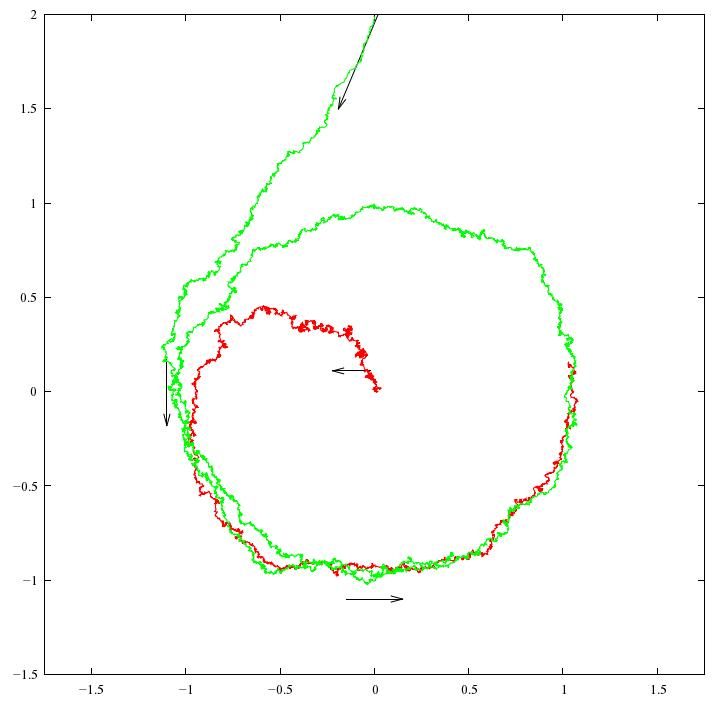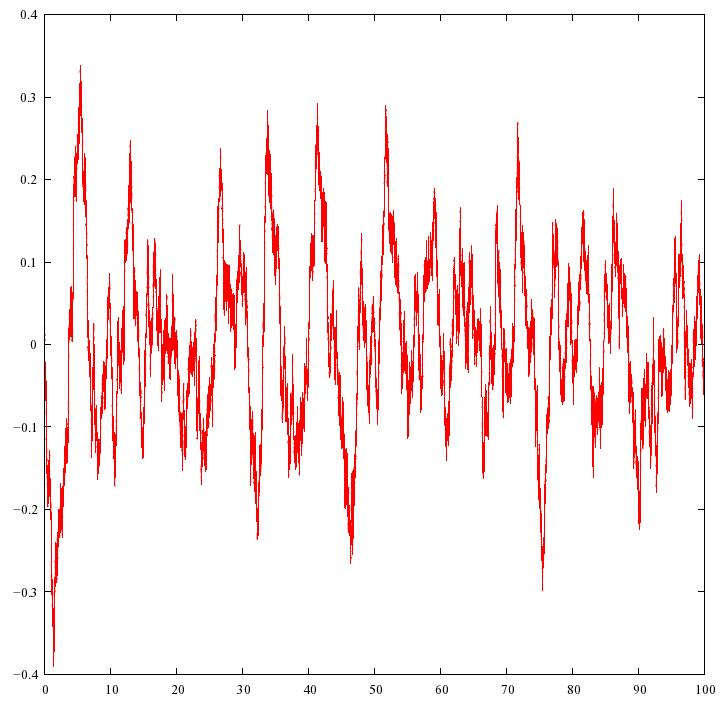# The Azimuth Project Hopf bifurcation (changes)

Showing changes from revision #6 to #7: Added | Removed | Changed

# Contents

## Idea

The Hopf bifurcation is a catastrophe in which as one gradually changes the parameters in an ordinary differential equation, a fixed point suddenly changes to a limit cycle:

This plays an important role in models of the El Nino, quantitative ecology, and many other subjects. In many of these cases it is interesting to add noise to the differential equation in question, obtaining a stochastic differential equation.

## Example

It is easiest to understand the idea by considering an example. There are various differential equations that exhibit a Hopf bifurcation, but here’s the simplest:

(1)$\frac{d x}{d t} = -y + \beta x - x (x^2 + y^2)$
(2)$\frac{d y}{d t} = \; x + \beta y - y (x^2 + y^2)$

Here $x$ and $y$ are functions of time, $t$, so these equations describe a point moving around on the plane. It’s easier to see what’s going on in polar coordinates:

(3)$\frac{d r}{d t} = \beta r - r^3$
(4)$\frac{d \theta}{d t} = 1$

The angle $\theta$ goes around at a constant rate while the radius $r$ does something more interesting. When $\beta \le 0$, you can see that any solution spirals in towards the origin. Or, if it starts at the origin, it stays there. So, we call the origin a stable equilibrium equilibrium.

Here’s a typical solution for $\beta = -1/4$, drawn as a curve in the $x y$ plane. As time passes, the solution spirals in towards the origin:The equations are more interesting for $\beta \gt 0$. Then $dr/dt = 0$ whenever

(5)$\beta r - r^3 = 0$

This has two solutions, $r = 0$ and $r = \sqrt{\beta}$. Since $r = 0$ is a solution, the origin is still an equilibrium. But now it’s not stable: if $r$ is between $0$ and $\sqrt{\beta}$, we’ll have $\beta r - r^3 \gt 0$, so our solution will spiral out, away from the origin and towards the circle $r = \sqrt{\beta}$. So, we say the origin is an unstable equilibrium. On the other hand, if $r$ starts out bigger than $\sqrt{\beta}$, our solution will spiral in towards that circle.

Here’s a picture of two solutions for $\beta = 1$:The red solution starts near the origin and spirals out towards the circle $r = \sqrt{\beta}$. The green solution starts outside this circle and spirals in towards it, soon becoming indistinguishable from the circle itself. So, this equation describes a system where $x$ and $y$ quickly settle down to a periodic oscillating behavior.

Since solutions that start anywhere near the circle $r = \sqrt{\beta}$ will keep going round and round getting closer to this circle, it’s called a "stable limit cycle".

This is what the Hopf bifurcation is all about: we’ve got a dynamical system that depends on a parameter, and as we change this parameter, a stable fixed point become unstable, and a stable limit cycle forms around it.

This isn’t quite a mathematical definition yet. If you want something a bit more precise, try:

## Stochastic version

The Hopf bifurcation is also important in models of the El Ni&ntilde;o / Southern Oscillation? or ENSO. However, the idea needs to be adapted to be useful. In the Hopf bifurcation described above, the system settles down into an orbit very close to the limit cycle, which is perfectly periodic. The ENSO cycle is only roughly periodic:

The time between El Niños varies between 3 and 7 years, averaging around 4 years. There can also be two El Niños without an intervening La Niña, or vice versa. One can try to explain this in various ways.

One very simple, general idea to add random noise to whatever differential equation we were using to model the ENSO cycle, obtaining a so-called stochastic differential equation: a differential equation describing a random process. Richard Kleeman discusses this idea in Tim Palmer’s book:

Kleeman mentions three general theories for the irregularity of the ENSO. They all involve the idea of separating the weather into "modes" — roughly speaking, different ways that things can oscillate. Some modes are slow and some are fast. The ENSO cycle is defined by the behavior of certain slow modes, but of course these interact with the fast modes. So, there are various options:

1. Perhaps the relevant slow modes interact with each other in a chaotic way.

2. Perhaps the relevant slow modes interact with each other in a non-chaotic way, but also interact with chaotic fast modes, which inject noise into what would otherwise be simple periodic behavior.

3. Perhaps the relevant slow modes interact with each other in a chaotic way, and also interact in a significant way with chaotic fast modes.

How can we get a simple model that illustrates the second option? Simple: take the model we just saw, and add some noise! This idea is discussed in detail here:

This paper is not about the ENSO cycle, but another one, which is often nicknamed the AMO. That’s not important here; all we are trying to explain here matters are the equations for a Hopf bifurcation with noise:

(6)$\frac{d x}{d t} = -y + \beta x - x (x^2 + y^2) + \lambda \frac{d W_1}{d t}$
(7)$\frac{d y}{d t} = \; x + \beta y - y (x^2 + y^2) + \lambda \frac{d W_2}{d t}$

They’re the same as before, but with some new extra terms at the end: that’s the noise.

This could easily get a bit technical, but click on the links for more information. $W_1$ and $W_2$ are two independent Wiener processes, so they describe Brownian motion in the $x$ and $y$ coordinates. When we differentiate a Wiener process we get white noise. So, we’re adding some amount of white noise to the equations we had before, and the number $\lambda$ says precisely how much. That means that $x$ and $y$ are no longer specific functions of time: they’re random functions, also known as stochastic processes.

If $\beta = 1$ and $\lambda = 0.1$, here are some typical solutions:They look similar to the solutions we saw before for $\beta = 1$, but now they have some random wiggles added on.

(You may be wondering what this picture really shows. After all, we said the solutions were random functions of time, not specific functions. But it’s tough to draw a "random function". So, to get one of the curves shown above, we randomly choose a function according to some rule for computing probabilities, and draw that.)

If we turn up the noise, our solutions get more wiggly. If $\beta = 1$ and $\lambda = 0.3$, they look like this:In these examples, $\beta \gt 0$, so we would have a limit cycle if there weren’t any noise — and you can see that even with noise, the solutions approximately tend towards the limit cycle. So, we can use an equation of this sort to describe systems that oscillate, but in a somewhat random way.

But now comes the really interesting part: suppose $\beta \le 0$. Then we’ve seen that without noise, there’s no limit cycle: any solution quickly spirals in towards the origin. But with noise, something a bit different happens. If $\beta = -1/4$ and $\lambda = 0.1$ we get a picture like this:We get irregular oscillations even though there’s no limit cycle! Roughly speaking, the noise keeps knocking the solution away from the stable fixed point at $x = y = 0$, so it keeps going round and round, but in an irregular way. It may seem to be spiralling in, but if we waited a bit longer it would get kicked out again.

This is a lot easier to see if we plot just $x$ as a function of $t$. Then we can run our solution for a longer time without the picture becoming a horrible mess:If you compare this with the ENSO cycle, you’ll see they look roughly similar:

That’s nice. Of course it doesn’t prove that a model based on a Hopf bifurcation plus noise is "right" — indeed, we don’t really have a model until we’ve chosen variables for both $x$ and $y$. But it suggests that a model of this sort could be worth studying.

To see how the Hopf bifurcation plus noise is applied to climate cycles, start with the paper by Dijkstra, Frankcombe and von der Heydt. To see it applied to the El Niño-Southern Oscillation, start with Section 6.3 of the ENSO theory paper, and then dig into the many references. Here it seems a model with $\beta \gt 0$ may work best. If so, noise is not required to keep the ENSO cycle going, but it makes the cycle irregular.

## Applications to quantitative ecology

You can see applications of the Hopf bifurcation and its stochastic version to predator-prey models here:

## Fokker-Planck equation

You can see applications of the Fokker-Planck equation to the equations we have been discussing:

$\frac{d x}{d t} = -y + \beta x - x (x^2 + y^2) + \lambda \frac{d W_1}{d t}$
$\frac{d y}{d t} = \; x + \beta y - y (x^2 + y^2) + \lambda \frac{d W_2}{d t}$

here: